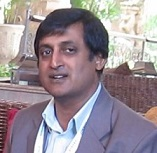Date: 10/03/2017

# Low power VLSI circuit modeling techniques

Date: 10/03/2017
Low power VLSI circuit modeling techniques

In this decade there is huge demand for low power VLSI semiconductor chips. In order to achive low power, power consumption should be minimized at CMOS MOSFET level. In this article, various techniques which are available for minimizing the power consumption at different abstraction levels are discussed in detail. With the help of this article, VLSI design engineers can pick the right methodology with suitable process techniques to reach their required goal.

Introduction

VLSI Integrated circuits are evolved about four decades ago. VLSI chip designers are still looking for enhancement in the performance of an integrated circuit by accomplishing the less area, low power utilization. Scaling techniques has been evolved to achieve these criteria. While scaling the power and area, various challenges encounters those are leakage currents (sub threshold leakage, gate tunneling, hot electron effect, impact ionization, gate induced drain leakage current etc).

Keeping in mind all those performance affecting parameters, VLSI designers produced various design mythologies. There are various techniques to achieve low power design. All this techniques are discussed in detail in this article.

Sources of power consumption in CMOS devices

The total power consumption of CMOS VLSI device can be expressed using equation 1.

Ptotal= Pdynamic + Pshort-circuit + Pstatic1

A. Dynamic power consumptionDynamic power consumption is due to charging and discharging of parasitic capacitance.

Energy/transition = CL* VDD2

Power=( Energy/transition)*f*N = CL* VDD2*f*N

Where VDD is power supply, N is the Switching activity; f is the frequency of the signal

B. Short circuit power consumption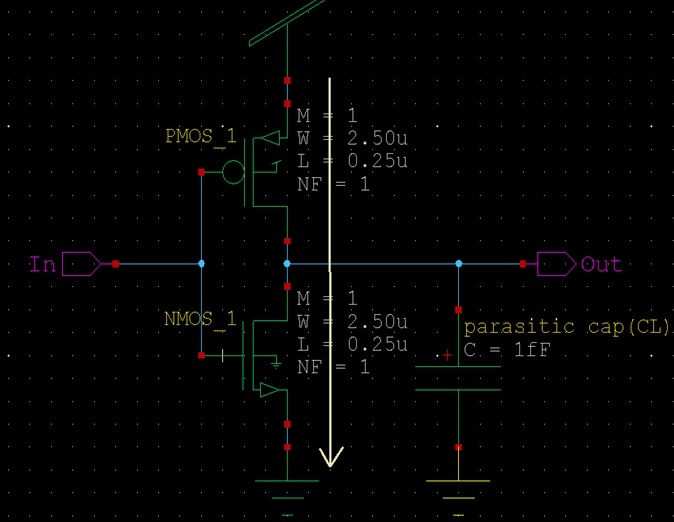Short circuit power consumption is due to the direct current path from VDD to GND while both PMOS and NMOS MOSFET devices are ON for short duration of time. Short circuit power consumption can be mathematically expressed as

Pshort_circuit= K*(VDD-2Vt)²*t*N*f

Where K is process technology parameter

Vt: threshold voltage

t:rise time (or) fall time

N: average number of transistors in the output stage

f: clock frequency

C. Static power consumption

Static power consumption is due to the leakage current in the MOSFET device.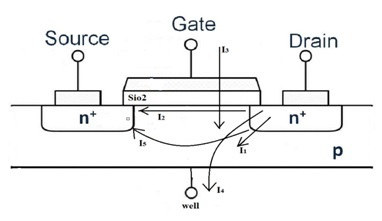I1: Reverse bias P-N junction leakage current

I2:Sub-Threshold leakage current

I3 :Gate tunneling current

I4 :Gate induced drain leakage

I5 :Channel punch through current

VLSI design methodology

VLSI Semiconductor IC performance relies upon conflicting parameters such as speed, power consumption, cost and production volume. These contemplations have prodded the improvement in distinct approaches in implementing IC ranging from high performance handcrafted design to fully programmable chip, medium to low performance designs.

Design methodology comparison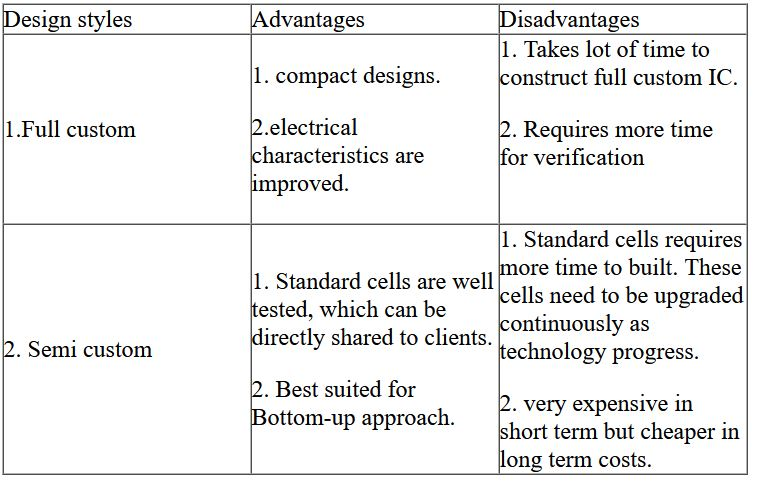Figure below shows VLSI IC design styles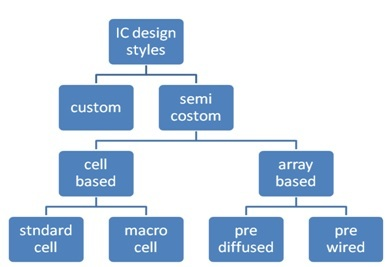Power reduction techniques at different abstraction levels

There is variety of techniques available to reduce the power consumption of the circuit at different abstraction levels those are mentioned below.

I. At circuit level

There are different VLSI circuits families that exist at this level of abstraction. Those are

Static CMOS

Figure below shows example of static CMOS MOSFET logic circuit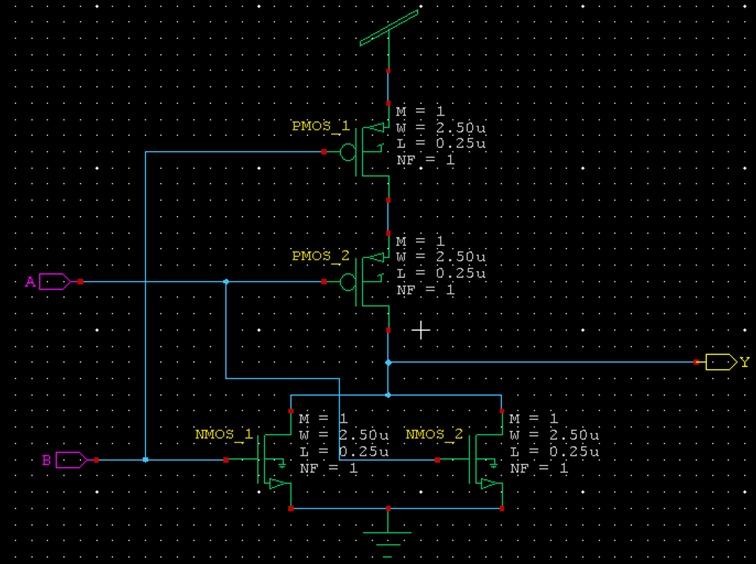In this logic, power consumption can be reduced by using:

a. Asymmetric gates

b. Switched gates

c. Multiple threshold voltage

2. Ratioed circuits

Pseudo NMOS logic can be used to reduce the power consumption of ratioed circuits. Figure below shows an example of Pseudo NMOS logic.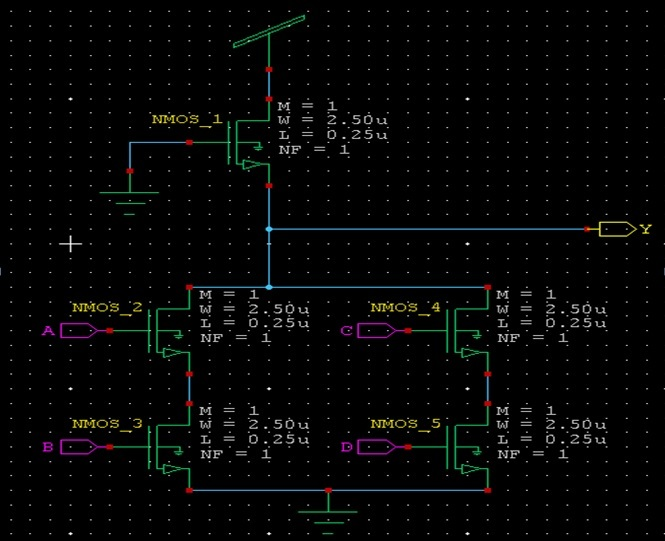Cascode voltage switch logic(CVSL)

CVSL logic offers low load capacitance on inputs, no static power consumption and also provides automatic complementary functions.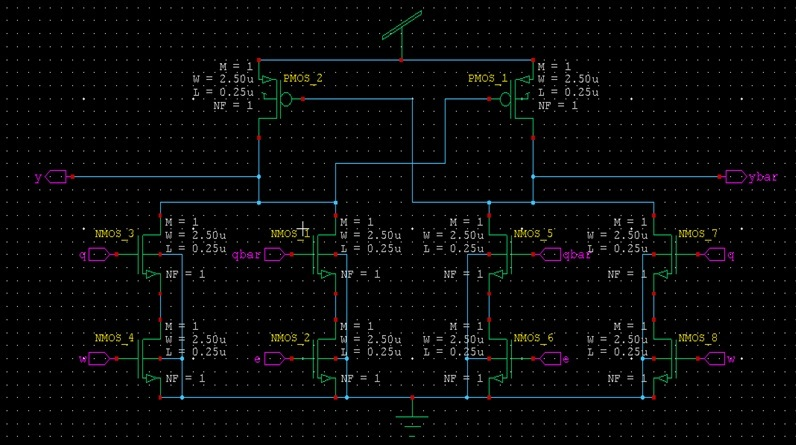Dynamic circuits: dynamic logic contains pre-charge and evaluation transistors which are driven by single clock, logic block is placed in between these transistors. Figure below shows the example of dynamic logic.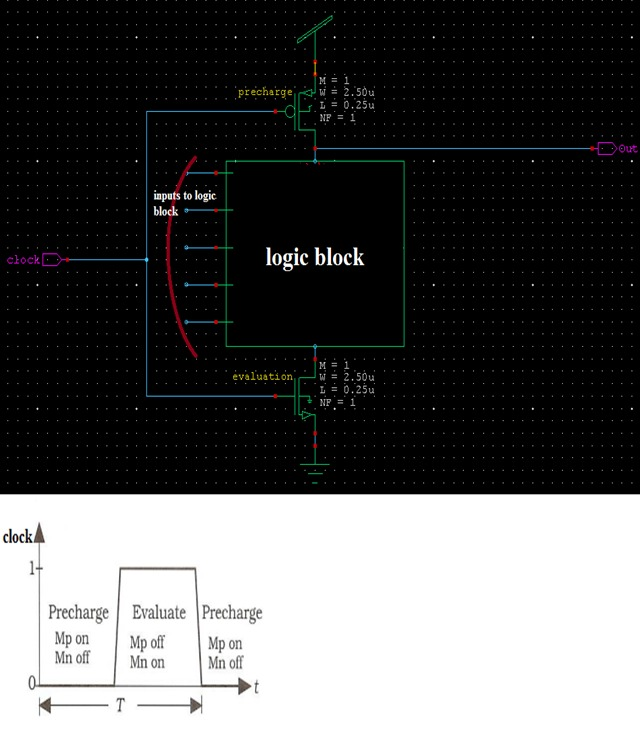Dynamic circuits can be implemented using following logics to optimize power.

(a). Domino logic: domino logic adds a buffer at the output of dynamic logic to avoid the affect of output high is pre-charged only with limited drive.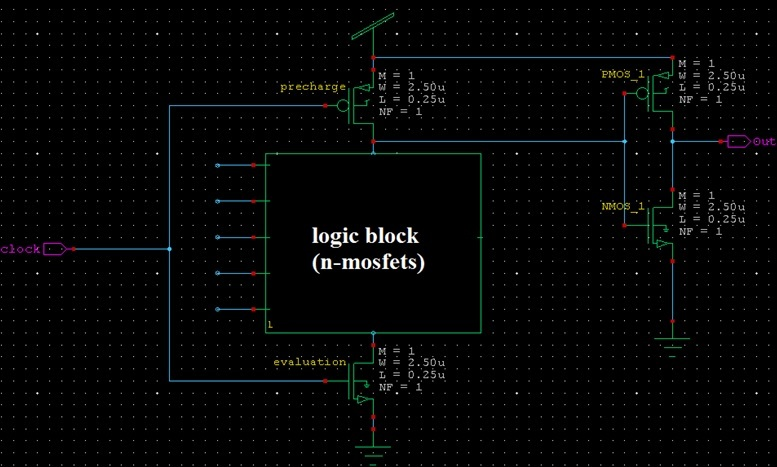Figure: Generic domino logic

Note: when several logic blocks are cascaded, one clock is not enough to drive entire circuit, solution for this problem is to use two phase clocks as shown below. Use of inverter at the output of dynamic logic can be avoided by using alternate n and p logic blocks.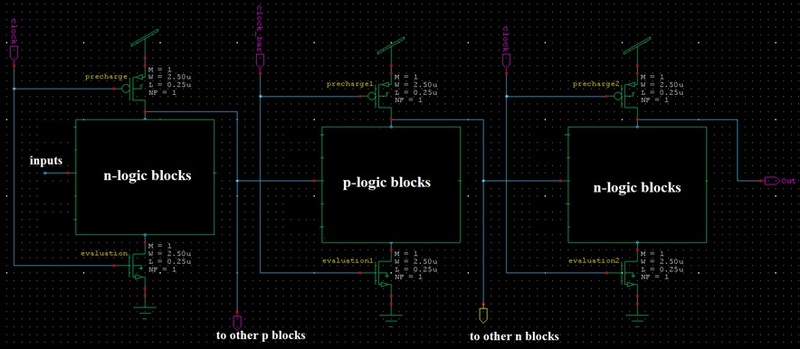NP dynamic logic sometime also called as NO Race(NORA)/zipper domino logic.

(b). Dual-rail domino logic: dual rail domino logic is not used for power optimization, because it doubles the number of gates.

Keepers logic: It resembles the same structure as domino, but it improves the performance by introducing keeper transistor at the output of dynamic logic. Keeper transistor will avoid the distribution of pre-charge charge over parasitic capacitance and NMOS array. Figure below shows the example of keeper logic.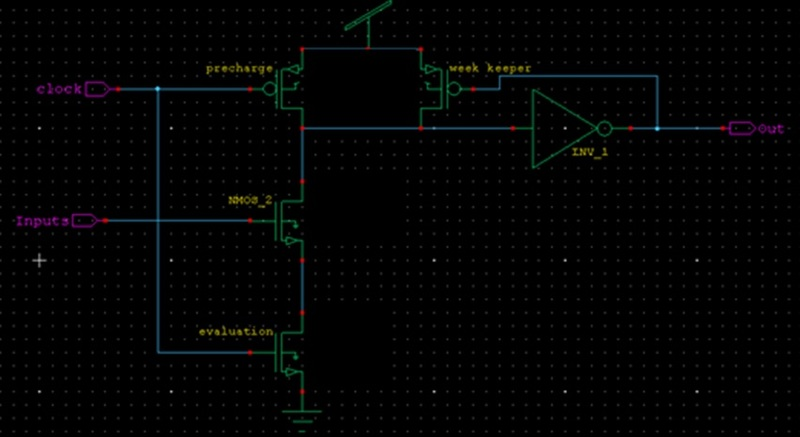(d). Multiple output domino logic (MODL):it enhance the performance by making use of intermediate nodes to form new outputs. But extra circuit may results to slow down the main output. Figure below shows the example of MODL logic.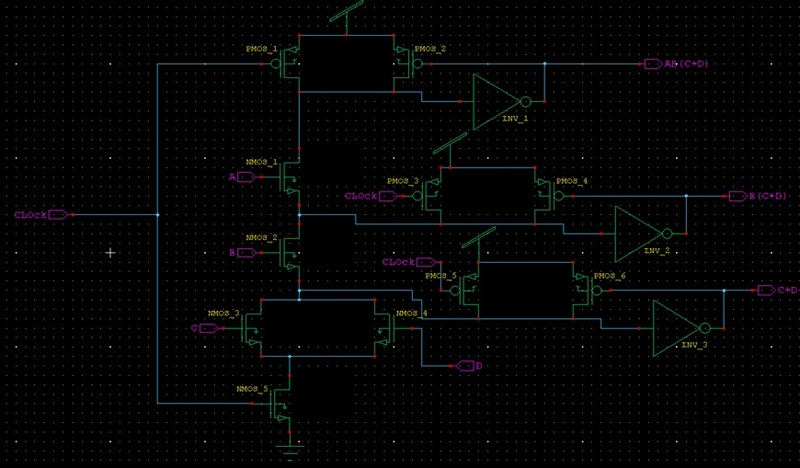Pass transistor logic: This type of logic circuits can be implemented using CMOS with transmission gates and complementary pass transistor logic for power optimization.

A. Complementary pass transistor (CPL) is a static gate, because its outputs are connected either to VDD or gnd via low resistance path. This logic facilitates the design of library of gates. Disadvantage is that extra circuit is required to generate differential signals.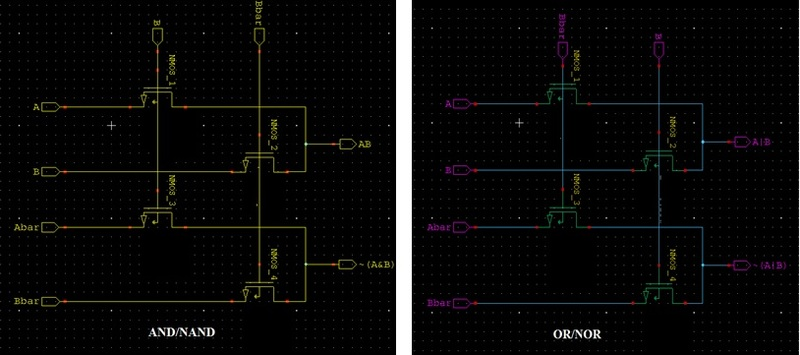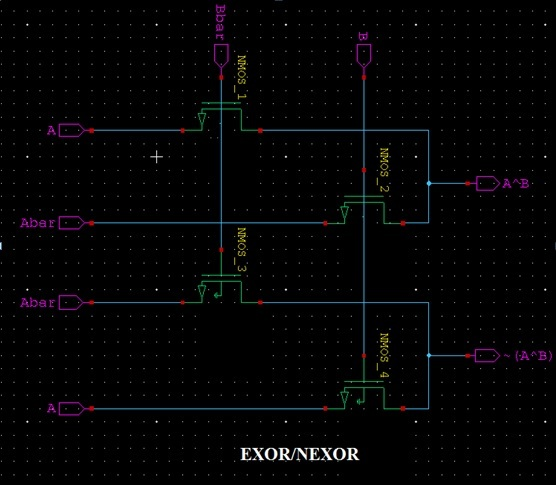B. CMOS with transmission gates: this design enables rail to rail swing. Usually efficient multiplexer designs are implemented using this logic.figure below shows the example of 2:1 mux implemented using this logic.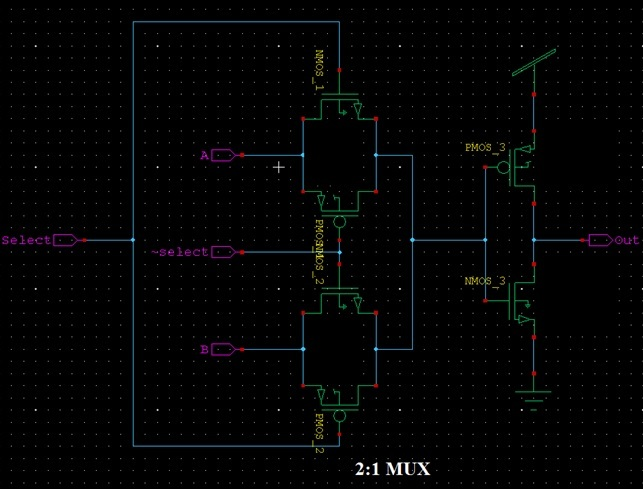Differential logic: this logic circuits can be implemented using following logics for power optimization.

Differential split level: The differential split-level (DSL) CMOS circuit technique has about 10X shorter propagation delay as compared to conventional CMOS in the same process, but at the cost of static power dissipation. DSL can be used in a conventional process in combination with conventional as well as other CMOS techniques on the same chip to combine fast subnanosecond and slower circuitry. Differential split-level CMOS logic is a circuit technique which allows, in any given technology, shorter channel length owing to a reduced drain-source voltage.

For more information refer differential split-level logic for subnanospeed speeds presented by L.C.M.G Pfennings, IEEE digital library.

Cascade non threshold logic: In this paper, two novel static CMOS logic circuits are proposed, analyzed, and partly chip-tested. First, the CMOS nonthreshold logic (CMOS NTL) is shown to be the fastest logic of the various static CMOS logic circuits. It has a simple logic construction style. The other new CMOS logic. The CMOS cascode nonthreshold logic (CMOS CNTL), is also shown to have a high speed performance. It offers a suitable trade-off between the high speed characteristics of the NTL circuits and the low power characteristics of the cascode CMOS circuits. We believe that these two new hgh-speed logic circuits will extend the application regime of digital CMOS IC's.

Reference: CMOS Nonthreshold Logic (NTL) and Cascode Nonthreshold Logic (CNTL) for High-speed Applications by jinn-shyan wang, IEEE digital library

Sample set differential logic(SSDL): It modifies the dual rail domino logic by adding a clocked latching sense amplifier. This kind of circuits are used for high speed operation. This kind of circuits suffers from static power consumption. This problem can be avoid using the concept of switched output differential structute(SODS).

Reference: Sample set differential logic(SSDC) for complex high-speed VLSI by T.A Grotjohn, IEEE digital library.

Enable/Disable CMOS differential logic(ECDL)

Latched CMOS differential logic(LCDL)

Differntial current switch logic (DCSL)

II. At logic level: Many optimization techniques are available at this level, those are:

If it is combitional logic circuit: following process should undergo for optimization

*Dont care optimization: circuits at multilevel are optimized by two-level minimization with appropriate dont care sets. The logic circuit structure may imply that some combination on the inputs of a given logic gate never occur, these combinations are called as satisfiability dont care sets(SDC) of the gate. Similarly there can be few input combinations for which output valve of the gate is not used in computation of any of the output of the circuit, these set of combinations are known as observability dont care sets(ODC).These dont care sets are used for area minimization and switching activity minimization at output of the logic gate.

*path balancing: path balancing is a solution to the problem that aries when there is no equal delay between the inputs of the logic block. When there is no equal delay, output of the logic block will contain spurious tone. Path balancing can be achieved through re-structuring the logic circuit, as depicted in the figure below.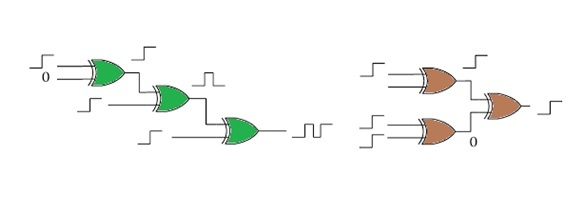* Logic factorization: A Primary means of technology optimization is the factoring of logic expression. For example consider an expression f=xy+xz+wy+wz this eqation can be factorized into (x+w)(y+z), which results reduced number of transistor count which intern reduces power consumption. Figure below shows the expression is implemented using gates without factorization.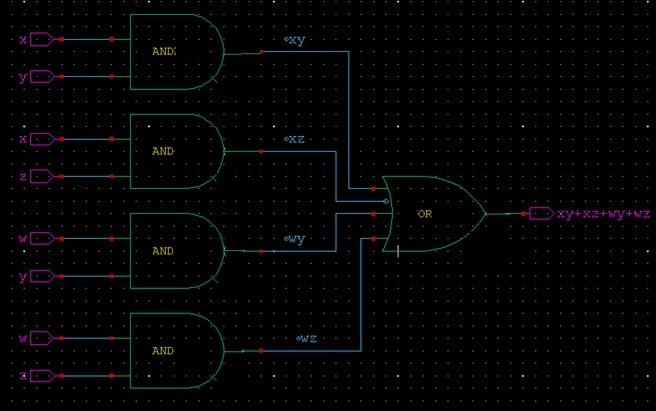Figure: logic implemented without factorization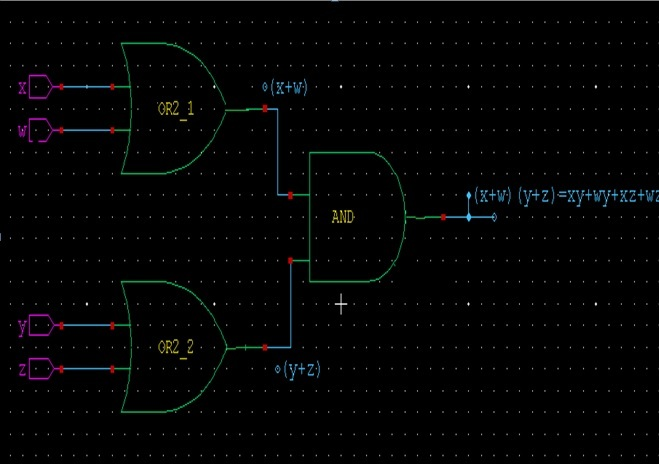Figure: reduced logic after factorization.

If it is a sequential logic: optimization should undergo following process

Encoding

Retiming

Pre- computation

III. At gate level: optimization techniques available at this level are

Technology mapping: technology mapping involves the optimal implementation of a Boolean function using gates from a given library. It serves as the final step in the logic synthesis pipeline. Output of the nodes of the logic circuit mapped to library gates under the cost function of load capacitance multiplied by switching activity. To minimize power dissipation, high switching activity points are either hidden within gates or driven by smaller gates. Minimum power realization under zero delay model can be obtained using dynamic programming.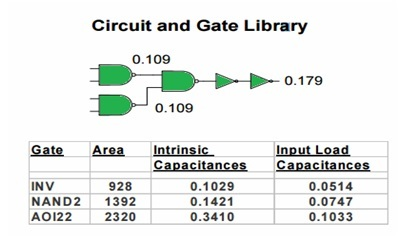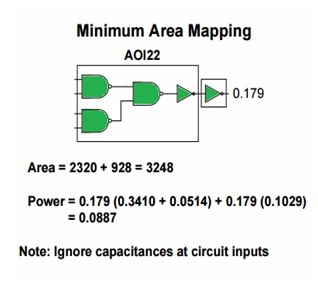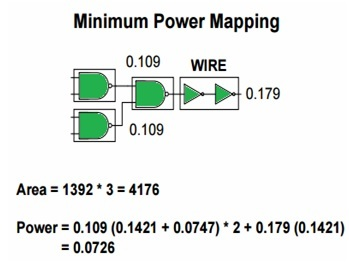Phase assignment : phase assignments inverts the inputs to an operation and, at the same time, also inverting the output. This transformation reduces power in the following ways. First, because this transformation adds inverts on nets that previously did not have inverters, it creates opportunity for several other transformations:two inverters next to each other can be merged and removed, and an inverter at the output of the gate may be absorbed into the gate using a composite gate from the library. Second, it can be used to remove inverters from high-activity nets and move then to low-activity nets.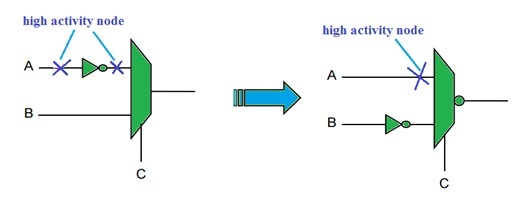Glitching power

IV. At RTL level: Optimization techniques available at this level are

signal gating

data path re-ordering

memory partition

V. At architectural level: optimization techniques available at this level are

parallel processing

pipeline processing

retiming

power management

bus segmentation

frequency reduction

VI. At system level: optimizing a circuit at this level should undergo following process

circuit portioning

node clustering

floor planning

placement

global routing

detailed routing

transistor and gate sizing

transistor re-ordering

super buffer design

wire sizing, driver sizing and buffer insertion

clock tree generation

power distribution

Author: Srinivasa Reddy N